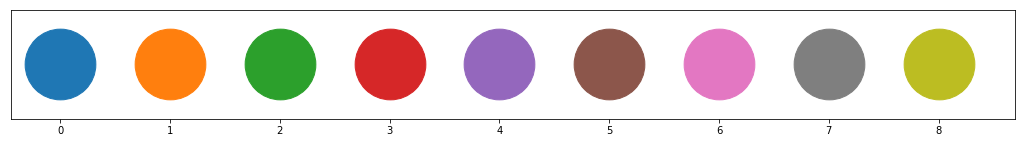# Classification of Marbles¶

In :
%matplotlib inline

In :
import os
import zipfile
import matplotlib.pyplot as plt
import numpy as np
import pandas as pd

from IPython.display import display, clear_output, Image, HTML

In :
HTML("""
<h2>Machine Learning in Action</h2>
<video width="640" height="360" controls>
<source src="http://files.point-8.de/mp4/point8_i40_demonstrator.mp4" type="video/mp4">
</video>
""")

Out:

## Machine Learning in Action

In this example we are going to use machine learning to automatically sort marbles by color. We only have RGB values from a light sensor as our raw data. The data was taken from nine different types of marbles using a sampling rate of 20 milliseconds while rotating each marbles.

Our task is to set up a machine learning workflow. Let’s have a short overview on the basic steps of machine learning. We will see that there is no magic behind it. The overall workflow is:

The overall workflow has been taken as an iterative process. The scikit-learn package provides the relevant algorithms and other tools.

Let’s dig into the data!!!

## Data Import and Preparation¶

The data preparation steps can take most of the time of the full workflow: In real world data, information is often missing, sanity checks have to be performed, data sets have to be joined from different sources and much more.

This function will help us to import the raw data - a list of tuples with color values ([(R,G,B),(R,G,B),(R,G,B)...]) for each type.

In :
def parse_lines(lines):
""" Parse strings of marble data"""
lines = lines[2:-2]
rows = [d.split(', ') for d in lines.split('), (')]
data = [[int(v.replace(')][(', '')) for v in r] for r in rows]
return pd.DataFrame(data)[[0, 1, 2]]


We import the raw data and create a list dfs containing a pandas.DataFrame for each file.

In :
data = []

files = [
'blue-white-glass.data',
'cyan-glass.data',
'glass-blue.data',
'glass-green.data',
'glass-red.data',
'glass-yellow.data',
'planet-black-blue.data',
'planet-green.data',
'planet-ocean.data',
]

dfs = []

for i, fname in enumerate(files):

with zipfile.ZipFile(f'../.assets/data/marbles/{fname}.zip', 'r') as zipf:
with zipf.open(f'{fname}', 'r') as infile:
dfs.append(parse_lines(content))

Load data 0: blue-white-glass.data


So far, we have a numerical index and no column names. Here we set the column names.

In :
for df in dfs:
df.columns=['R','G','B']


We define a color code that we know what we will talk about and can use in plots accordingly.

In :
plt.figure(figsize=(18,2))
for i in range(9):
plt.scatter([i],,s=5000)
plt.xticks(np.linspace(0,8,9))
plt.yticks([])
plt.show()In :
display(Image('../.assets/data/marbles/Murmeln.png'))